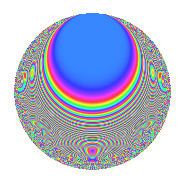# Properties

 Label 670.2.eLevel 670 Weight 2 Character orbit e Rep. character $$\chi_{670}(171,\cdot)$$ Character field $$\Q(\zeta_{3})$$ Dimension 48 Newforms 10 Sturm bound 204 Trace bound 9

# Related objects

## Defining parameters

 Level: $$N$$ = $$670 = 2 \cdot 5 \cdot 67$$ Weight: $$k$$ = $$2$$ Character orbit: $$[\chi]$$ = 670.e (of order $$3$$ and degree $$2$$) Character conductor: $$\operatorname{cond}(\chi)$$ = $$67$$ Character field: $$\Q(\zeta_{3})$$ Newforms: $$10$$ Sturm bound: $$204$$ Trace bound: $$9$$ Distinguishing $$T_p$$: $$3$$, $$7$$, $$11$$

## Dimensions

The following table gives the dimensions of various subspaces of $$M_{2}(670, [\chi])$$.

Total New Old
Modular forms 212 48 164
Cusp forms 196 48 148
Eisenstein series 16 0 16

## Trace form

 $$48q + 2q^{2} + 4q^{3} - 24q^{4} - 4q^{5} - 4q^{6} - 4q^{7} - 4q^{8} + 64q^{9} + O(q^{10})$$ $$48q + 2q^{2} + 4q^{3} - 24q^{4} - 4q^{5} - 4q^{6} - 4q^{7} - 4q^{8} + 64q^{9} - 2q^{12} - 4q^{13} + 12q^{14} - 24q^{16} - 6q^{17} + 4q^{18} - 6q^{19} + 2q^{20} + 2q^{21} - 40q^{22} + 12q^{23} + 8q^{24} + 48q^{25} + 4q^{27} - 4q^{28} + 6q^{29} + 2q^{30} + 8q^{31} + 2q^{32} - 4q^{33} - 10q^{34} - 4q^{35} - 32q^{36} - 8q^{37} + 18q^{38} + 48q^{39} + 6q^{41} + 8q^{42} - 4q^{43} - 8q^{45} - 18q^{46} - 8q^{47} - 2q^{48} - 42q^{49} + 2q^{50} - 30q^{51} + 8q^{52} - 80q^{53} + 8q^{54} + 12q^{55} - 6q^{56} + 58q^{57} - 24q^{58} - 4q^{59} - 28q^{61} + 16q^{62} - 40q^{63} + 48q^{64} + 8q^{65} - 16q^{66} - 64q^{67} + 12q^{68} - 26q^{69} + 4q^{70} + 24q^{71} - 8q^{72} - 34q^{73} - 8q^{74} + 4q^{75} + 12q^{76} - 16q^{77} - 12q^{78} - 16q^{79} + 2q^{80} + 144q^{81} + 8q^{82} + 2q^{84} - 12q^{87} + 20q^{88} - 116q^{89} - 24q^{91} - 24q^{92} + 88q^{93} - 12q^{94} - 12q^{95} - 4q^{96} - 34q^{97} + 34q^{98} - 48q^{99} + O(q^{100})$$

## Decomposition of $$S_{2}^{\mathrm{new}}(670, [\chi])$$ into irreducible Hecke orbits

Label Dim. $$A$$ Field CM Traces $q$-expansion
$$a_2$$ $$a_3$$ $$a_5$$ $$a_7$$
670.2.e.a $$2$$ $$5.350$$ $$\Q(\sqrt{-3})$$ None $$-1$$ $$-6$$ $$-2$$ $$2$$ $$q+(-1+\zeta_{6})q^{2}-3q^{3}-\zeta_{6}q^{4}-q^{5}+\cdots$$
670.2.e.b $$2$$ $$5.350$$ $$\Q(\sqrt{-3})$$ None $$-1$$ $$-6$$ $$2$$ $$-4$$ $$q+(-1+\zeta_{6})q^{2}-3q^{3}-\zeta_{6}q^{4}+q^{5}+\cdots$$
670.2.e.c $$2$$ $$5.350$$ $$\Q(\sqrt{-3})$$ None $$-1$$ $$2$$ $$-2$$ $$5$$ $$q+(-1+\zeta_{6})q^{2}+q^{3}-\zeta_{6}q^{4}-q^{5}+\cdots$$
670.2.e.d $$2$$ $$5.350$$ $$\Q(\sqrt{-3})$$ None $$1$$ $$2$$ $$-2$$ $$-2$$ $$q+(1-\zeta_{6})q^{2}+q^{3}-\zeta_{6}q^{4}-q^{5}+(1+\cdots)q^{6}+\cdots$$
670.2.e.e $$4$$ $$5.350$$ $$\Q(\sqrt{-3}, \sqrt{193})$$ None $$-2$$ $$4$$ $$-4$$ $$-4$$ $$q-\beta _{2}q^{2}+q^{3}+(-1+\beta _{2})q^{4}-q^{5}+\cdots$$
670.2.e.f $$4$$ $$5.350$$ $$\Q(\sqrt{-2}, \sqrt{-3})$$ None $$-2$$ $$4$$ $$-4$$ $$-4$$ $$q-\beta _{1}q^{2}+(1-\beta _{3})q^{3}+(-1+\beta _{1})q^{4}+\cdots$$
670.2.e.g $$6$$ $$5.350$$ 6.0.954288.1 None $$3$$ $$-2$$ $$6$$ $$-2$$ $$q+(1-\beta _{3})q^{2}+\beta _{4}q^{3}-\beta _{3}q^{4}+q^{5}+\cdots$$
670.2.e.h $$6$$ $$5.350$$ 6.0.2696112.1 None $$3$$ $$2$$ $$6$$ $$2$$ $$q+\beta _{3}q^{2}-\beta _{2}q^{3}+(-1+\beta _{3})q^{4}+q^{5}+\cdots$$
670.2.e.i $$8$$ $$5.350$$ $$\mathbb{Q}[x]/(x^{8} - \cdots)$$ None $$-4$$ $$8$$ $$8$$ $$0$$ $$q+(-1+\beta _{2})q^{2}+(1+\beta _{3})q^{3}-\beta _{2}q^{4}+\cdots$$
670.2.e.j $$12$$ $$5.350$$ $$\mathbb{Q}[x]/(x^{12} - \cdots)$$ None $$6$$ $$-4$$ $$-12$$ $$3$$ $$q-\beta _{5}q^{2}-\beta _{2}q^{3}+(-1-\beta _{5})q^{4}-q^{5}+\cdots$$

## Decomposition of $$S_{2}^{\mathrm{old}}(670, [\chi])$$ into lower level spaces

$$S_{2}^{\mathrm{old}}(670, [\chi]) \cong$$ $$S_{2}^{\mathrm{new}}(67, [\chi])$$$$^{\oplus 4}$$$$\oplus$$$$S_{2}^{\mathrm{new}}(134, [\chi])$$$$^{\oplus 2}$$$$\oplus$$$$S_{2}^{\mathrm{new}}(335, [\chi])$$$$^{\oplus 2}$$